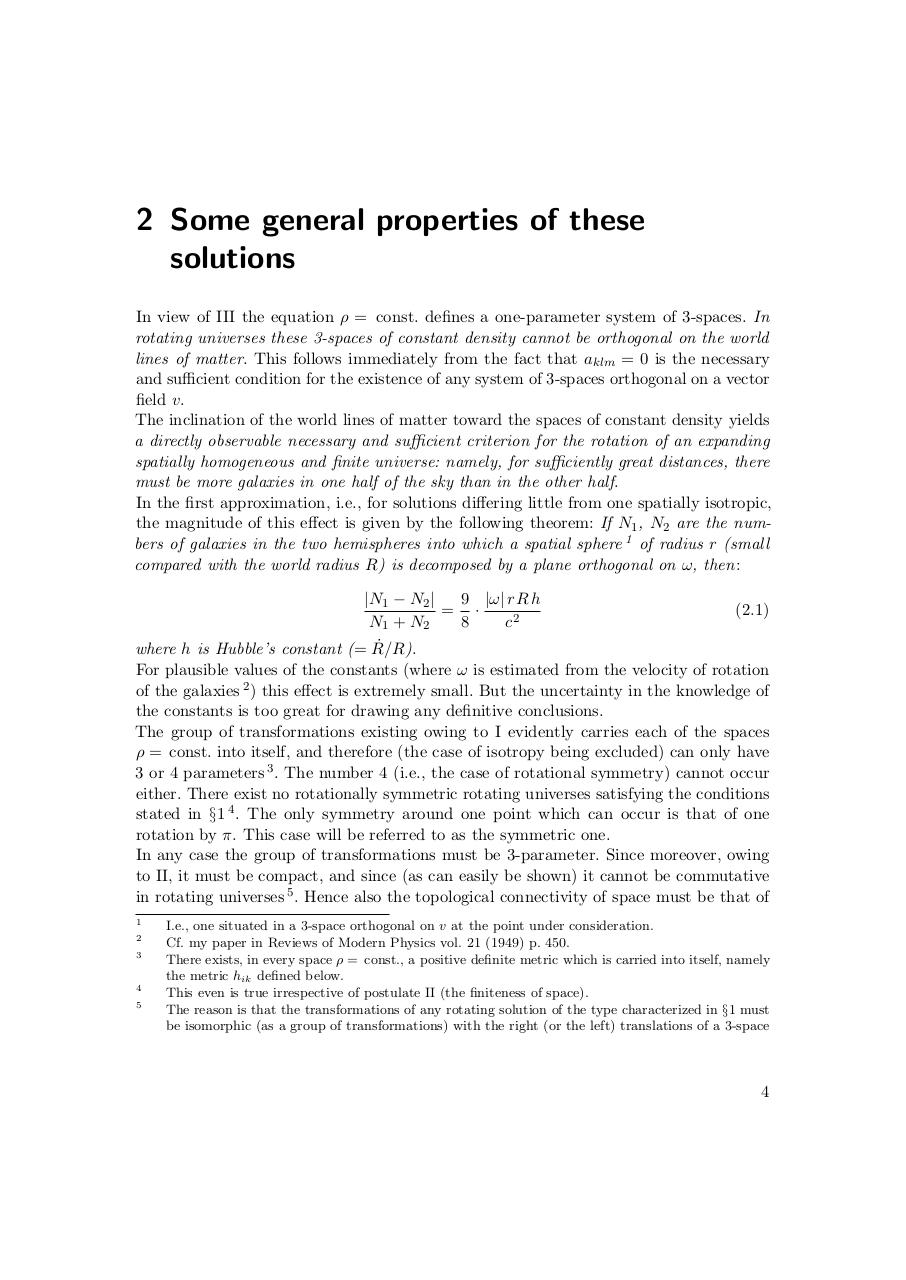# Kurt Gödel. Rotating Universes In General Relativity Theory.pdfPage 1 2 3 4 5 6 7 8 9 10 11

#### Text preview

2 Some general properties of these
solutions
In view of III the equation ρ = const. defines a one-parameter system of 3-spaces. In
rotating universes these 3-spaces of constant density cannot be orthogonal on the world
lines of matter. This follows immediately from the fact that aklm = 0 is the necessary
and sufficient condition for the existence of any system of 3-spaces orthogonal on a vector
field v.
The inclination of the world lines of matter toward the spaces of constant density yields
a directly observable necessary and sufficient criterion for the rotation of an expanding
spatially homogeneous and finite universe: namely, for sufficiently great distances, there
must be more galaxies in one half of the sky than in the other half.
In the first approximation, i.e., for solutions differing little from one spatially isotropic,
the magnitude of this effect is given by the following theorem: If N1 , N2 are the numbers of galaxies in the two hemispheres into which a spatial sphere 1 of radius r (small
compared with the world radius R) is decomposed by a plane orthogonal on ω, then:
|N1 − N2 |
9 |ω| r R h
= ·
N1 + N2
8
c2

(2.1)

where h is Hubble’s constant (= Ṙ/R).
For plausible values of the constants (where ω is estimated from the velocity of rotation
of the galaxies 2 ) this effect is extremely small. But the uncertainty in the knowledge of
the constants is too great for drawing any definitive conclusions.
The group of transformations existing owing to I evidently carries each of the spaces
ρ = const. into itself, and therefore (the case of isotropy being excluded) can only have
3 or 4 parameters 3 . The number 4 (i.e., the case of rotational symmetry) cannot occur
either. There exist no rotationally symmetric rotating universes satisfying the conditions
stated in §1 4 . The only symmetry around one point which can occur is that of one
rotation by π. This case will be referred to as the symmetric one.
In any case the group of transformations must be 3-parameter. Since moreover, owing
to II, it must be compact, and since (as can easily be shown) it cannot be commutative
in rotating universes 5 . Hence also the topological connectivity of space must be that of
1
2
3

4
5

I.e., one situated in a 3-space orthogonal on v at the point under consideration.
Cf. my paper in Reviews of Modern Physics vol. 21 (1949) p. 450.
There exists, in every space ρ = const., a positive definite metric which is carried into itself, namely
the metric hik defined below.
This even is true irrespective of postulate II (the finiteness of space).
The reason is that the transformations of any rotating solution of the type characterized in §1 must
be isomorphic (as a group of transformations) with the right (or the left) translations of a 3-space

4